Author: Oscar Cronquist Article last updated on October 01, 2019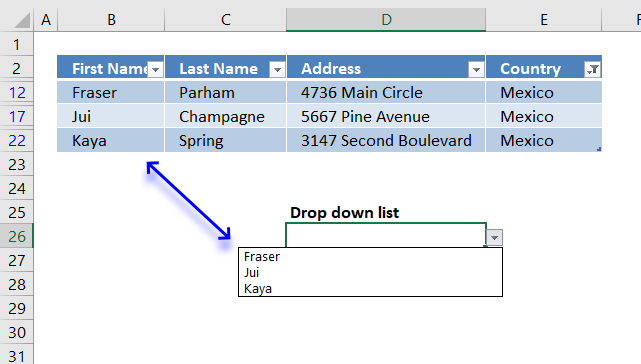This article demonstrates how to populate a drop down list with filtered values from an Excel defined Table.

The animated image below demonstrates how values (First Name) in a drop down list changes based on how the table is filtered (Country).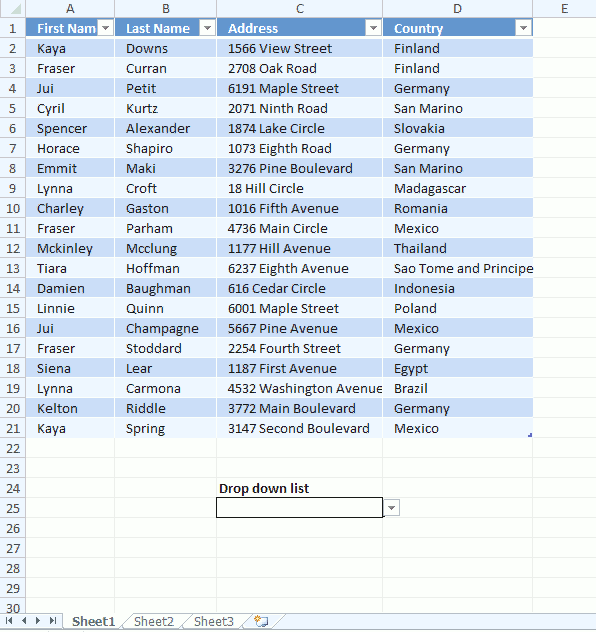### How to build this

First we need to insert a new worksheet, this new worksheet will contain a formula that extracts filtered values from the Excel defined Table.

The drop-down list contains a named range that will fetch the extracted values from the new worksheet.

#### Calculation worksheet

1. Press with right mouse button on on any worksheet tab at the very bottom of the Excel screen.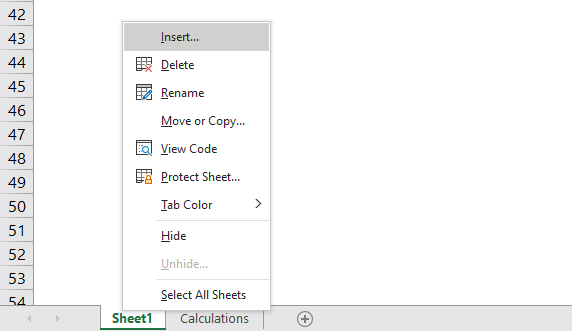2. Press with mouse on "Insert" to open a dialog box.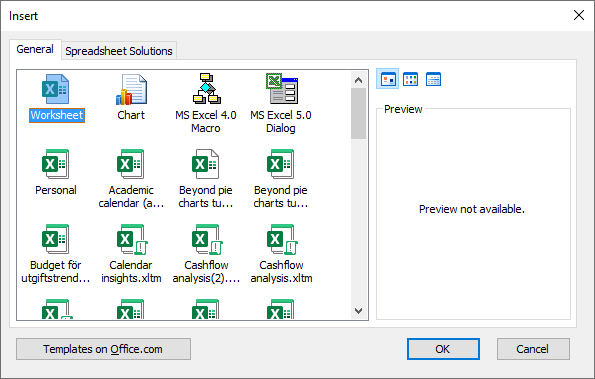3. Select "Worksheet" icon.
4. Press with left mouse button on OK button.

To rename the worksheet simply press with right mouse button on on the worksheet tab and then press with left mouse button on "Rename".

1.Type a new worksheet name and press Enter, I named it "Calculations".

### Formula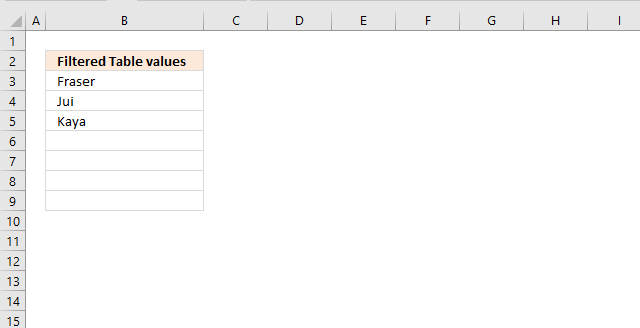I entered a header name in cell B2.

Formula in cell B3:

=IFERROR(INDEX(Table2[First Name], SMALL(IF(SUBTOTAL(3, OFFSET(Table2[First Name], MATCH(ROW(Table2[First Name]), ROW(Table2[First Name]))-1, 0, 1)), MATCH(ROW(Table2[First Name]), ROW(Table2[First Name])), ""), ROWS(\$A\$1:A1))), "")
1. Double press with left mouse button on cell B3.
2. Paste above array formula, shortcut CTRL + v.
3. Press and hold CTRL and SHIFT keys simultaneously
4. Press Enter once

The formula in the formula bar now looks like this: {=formula}
Don't enter these curly parentheses yourself, they appear automatically if you did the above steps correctly.

#### Explaining formula in cell B3

Step 1 - Create an array

The OFFSET function allows you to identify filtered values if you combine it with the SUBTOTAL function.

OFFSET(Table2[First Name], MATCH(ROW(Table2[First Name]), ROW(Table2[First Name]))-1, 0, 1)

becomes

OFFSET(Table2[First Name], {0; 1; 2; 3; 4; 5; 6; 7; 8; 9; 10; 11; 12; 13; 14; 15; 16; 17; 18; 19}, 0, 1)

and returns

{"Kaya"; "Fraser"; "Jui"; "Cyril"; "Spencer"; "Horace"; "Emmit"; "Lynna"; "Charley"; "Fraser"; "Mckinley"; "Tiara"; "Damien"; "Linnie"; "Jui"; "Fraser"; "Siena"; "Lynna"; "Kelton"; "Kaya"}.

This step may seem weird when you can just use a cell reference to the values, however, that won't work. This step is necessary in order to force the SUBTOTAL function to evaluate each value in the array.

Note, the OFFSET function is volatile meaning it is recalculated each time the workbook is recalculated. Extensive use may slow down your workbook considerably.

Step 2 - Identify filtered values

The SUBTOTAL function returns 0 (zero) if value is not visible and 1 if the value is visible.

SUBTOTAL(3, OFFSET(Table2[First Name], MATCH(ROW(Table2[First Name]), ROW(Table2[First Name]))-1, 0, 1))

becomes

SUBTOTAL(3, {"Kaya"; "Fraser"; "Jui"; "Cyril"; "Spencer"; "Horace"; "Emmit"; "Lynna"; "Charley"; "Fraser"; "Mckinley"; "Tiara"; "Damien"; "Linnie"; "Jui"; "Fraser"; "Siena"; "Lynna"; "Kelton"; "Kaya"})

and returns

{0; 0; 0; 0; 0; 0; 0; 0; 0; 1; 0; 0; 0; 0; 1; 0; 0; 0; 0; 1}

Step 3 - Return row numbers of filtered values

The IF function returns the corresponding row number of each filtered (visible) value and a blank for not visible values.

IF(SUBTOTAL(3, OFFSET(Table2[First Name], MATCH(ROW(Table2[First Name]), ROW(Table2[First Name]))-1, 0, 1)), MATCH(ROW(Table2[First Name]), ROW(Table2[First Name])), "")

becomes

IF({0; 0; 0; 0; 0; 0; 0; 0; 0; 1; 0; 0; 0; 0; 1; 0; 0; 0; 0; 1}, MATCH(ROW(Table2[First Name]), ROW(Table2[First Name])), "")

becomes

IF({0; 0; 0; 0; 0; 0; 0; 0; 0; 1; 0; 0; 0; 0; 1; 0; 0; 0; 0; 1}, {1; 2; 3; 4; 5; 6; 7; 8; 9; 10; 11; 12; 13; 14; 15; 16; 17; 18; 19; 20}, "")

and returns

{""; ""; ""; ""; ""; ""; ""; ""; ""; 10; ""; ""; ""; ""; 15; ""; ""; ""; ""; 20}

Step 4 - Extract the k-th smallest row number

The SMALL function extracts the k-th smallest number in a cell range or array.

SMALL(array, k)

SMALL(IF(SUBTOTAL(3, OFFSET(Table2[First Name], MATCH(ROW(Table2[First Name]), ROW(Table2[First Name]))-1, 0, 1)), MATCH(ROW(Table2[First Name]), ROW(Table2[First Name])), ""), ROWS(\$A\$1:A1))

becomes

SMALL({""; ""; ""; ""; ""; ""; ""; ""; ""; 10; ""; ""; ""; ""; 15; ""; ""; ""; ""; 20}, ROWS(\$A\$1:A1))

becomes

SMALL({""; ""; ""; ""; ""; ""; ""; ""; ""; 10; ""; ""; ""; ""; 15; ""; ""; ""; ""; 20}, 1)

and returns 10.

Step 5 - Return value based on row number

The INDEX function returns a value based on a row number (and column number if needed).

INDEX(Table2[First Name], SMALL(IF(SUBTOTAL(3, OFFSET(Table2[First Name], MATCH(ROW(Table2[First Name]), ROW(Table2[First Name]))-1, 0, 1)), MATCH(ROW(Table2[First Name]), ROW(Table2[First Name])), ""), ROWS(\$A\$1:A1)))

becomes

INDEX(Table2[First Name], 10)

becomes

INDEX({"Kaya"; "Fraser"; "Jui"; "Cyril"; "Spencer"; "Horace"; "Emmit"; "Lynna"; "Charley"; "Fraser"; "Mckinley"; "Tiara"; "Damien"; "Linnie"; "Jui"; "Fraser"; "Siena"; "Lynna"; "Kelton"; "Kaya"}, 10)

and returns "Fraser" in cell B4 which is the 10th value in the array.

The IFERROR function removes errors that will show when there are no more values to show.

#### Create named range

1. Go to tab "Formulas" on the ribbon.
2. Press with left mouse button on "Name Manager" button.
3. Press with left mouse button on "New.." button to open the Name Manager dialog box.
4. Name: Drop_down_list
5. Refers to: =INDIRECT("Calculations!\$B\$3:\$B\$"&SUMPRODUCT((Calculations!\$B:\$B<>"")*1)+1)
6. Press with left mouse button on OK!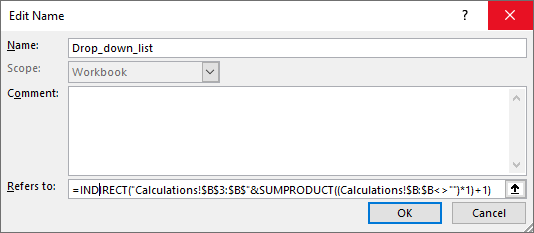7. Press with left mouse button on Close!

#### Explaining named range formulaThe formula used in name range Drop_down_list is dynamic and changes based on the number of values in column B in worksheet "Calculations.

Step 1 - Identify non empty cells in column B

The less and greater than signs combined checks if cells are empty or not.

Calculations!\$B:\$B<>""

becomes

{""; "Filtered Table values"; "Fraser"; "Jui"; "Kaya"; "" ... ""}<>""

and returns

{"FALSE";"TRUE";"TRUE";"TRUE"; "TRUE"; "FALSE"... ; "FALSE"}

It is not neccessary to check every cell in column B, if this calculation is  slow for you then use a smaller cell reference, for example: Calculations!\$B\$1:\$B\$1000<>""

Step 2 - Count non empty cells in column B

The SUMPRODUCT function returns a number representing the total number of non empty cells.

SUMPRODUCT((Calculations!\$B:\$B<>"")*1)

becomes

SUMPRODUCT({"FALSE";"TRUE";"TRUE";"TRUE"; "TRUE"; "FALSE"... ; "FALSE"}*1)

The SUMPRODUCT function can't sum boolean values (TRUE or FALSE) so we need to convert them to their numerical equivalents, this is done by multiplying with 1.

SUMPRODUCT({0; 1; 1; 1; 1; 0... ; 0})

and returns 4.

Step 3 - Create cell reference

The ampersand allows you to combine text with a calculation.

"Calculations!\$B\$3:\$B\$"&SUMPRODUCT((Calculations!\$B:\$B<>"")*1)+1

becomes

"Calculations!\$B\$3:\$B\$"&4+1

becomes

"Calculations!\$B\$3:\$B\$"&5

and returns

"Calculations!\$B\$3:\$B\$5"

Step 4 - Convert text to cell reference

The INDIRECT function lets you use the text as a cell reference.

INDIRECT("Calculations!\$B\$3:\$B\$"&SUMPRODUCT((Calculations!\$B:\$B<>"")*1)+1)

becomes

INDIRECT("Calculations!\$B\$3:\$B\$5")

and returns the values in cell range Calculations!\$B\$3:\$B\$5.

#### Create drop down list

1. Select cell D26
2. Go to tab "Data" on the riboon.
3. Press with left mouse button on "Data Validation" button.
4. Allow: List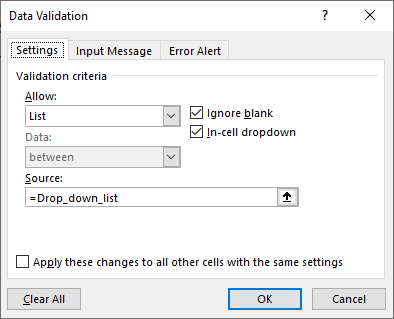5. Source: =Drop_down_list
6. Press with left mouse button on Ok

### Get the Excel file